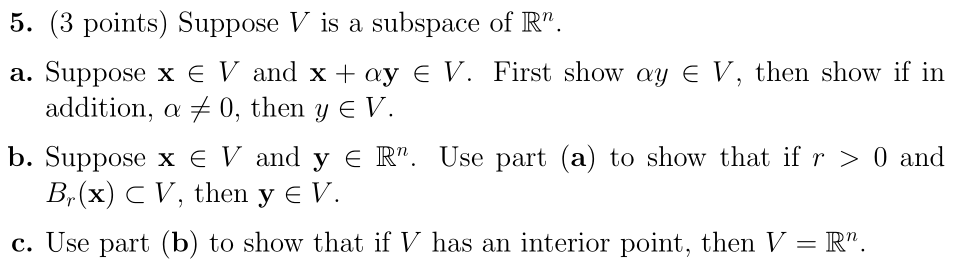Home / Answered Questions / Other / 5-3-points-suppose-v-is-a-subspace-of-r-a-suppose-x-e-v-and-x-ay-v-first-show-ay-e-v-then-show-if-in-aw578

# (Solved): 5. (3 Points) Suppose V Is A Subspace Of Râ€. A. Suppose X E V And X + Ay V. First Show Ay E V, The...5. (3 points) Suppose V is a subspace of Râ€. a. Suppose x e V and x + ay V. First show ay E V, then show if in addition, a + 0, then y EV. b. Suppose x E V and y ER". Use part (a) to show that if r > 0) and B,(x) CV, then y EV. c. Use part (b) to show that if V has an interior point, then V = Râ€.

We have an Answer from Expert